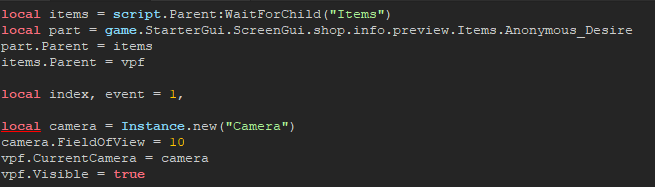# Help with Viewportframe

I need help fixing my viewportframe so it shows specific models in my items folder. In the script it only shows everything at once but I don’t wan that.
here is the code

``````local vpf = script.Parent
local items = script.Parent:WaitForChild("Items")
local children = items:GetChildren()

local index, event = 1, nil

local camera = Instance.new("Camera")
camera.FieldOfView = 10
vpf.CurrentCamera = camera
vpf.Visible = true

-- rotation

local function setRotationEvent(model)
local currentAngle = 0
local modelCF, modelSize = model:GetBoundingBox()

local rotInv = (modelCF - modelCF.p):inverse()
modelCF = modelCF * rotInv
modelSize = rotInv * modelSize
modelSize = Vector3.new(math.abs(modelSize.x), math.abs(modelSize.y), math.abs(modelSize.z))

local diagonal = 0
local maxExtent = math.max(modelSize.x, modelSize.y, modelSize.z)

if (maxExtent == modelSize.x) then
diagonal = math.sqrt(modelSize.y*modelSize.y + modelSize.z*modelSize.z)/2
elseif (maxExtent == modelSize.y) then
diagonal = math.sqrt(modelSize.x*modelSize.x + modelSize.z*modelSize.z)/2
else
diagonal = math.sqrt(modelSize.x*modelSize.x + modelSize.y*modelSize.y)/2
end

local minDist = (maxExtent/2)/tan + diagonal

return game:GetService("RunService").RenderStepped:Connect(function(dt)
currentAngle = currentAngle + 1*dt*40
camera.CFrame = modelCF * CFrame.fromEulerAnglesYXZ(0, math.rad(currentAngle), 0) * CFrame.new(0, 0, minDist + 3)
end)
end

local function setIndexActive(newIndex)
if (event) then
event:Disconnect()
children[index].Parent = items
end

index = newIndex
event = setRotationEvent(children[index])
children[index].Parent = vpf
end

--

setIndexActive(index)

vpf.InputBegan:Connect(function(input)
if (input.UserInputType == Enum.UserInputType.MouseButton1) then
end
end)
``````

and here is a video showing it

any model/part that is a ‘child’ of the viewport frame will be viewed.

``````local function setIndexActive(newIndex)
if (event) then
event:Disconnect()
children[index].Parent = items
end

index = newIndex
event = setRotationEvent(children[index])
children[index].Parent = vpf
end
``````

this function right here adds everything to your viewport frame, so if you don’t want to view everything at once then you’ll have to re-do this function and only add parts/models that you want to see.

``````local part = game.Workspace.SomePart
part.Parent = vfp
``````

So what part of the script will I have to change it in order for me to only show certain models?
What am I gonna change it to?

``````local function setIndexActive(newIndex)
if (event) then
event:Disconnect()
children[index].Parent = items
end

index = newIndex
event = setRotationEvent(children[index])
children[index].Parent = vpf
end
``````

you would change this function into

``````local function addChildren()
--parent parts or models to the viewport frame here.
local somePart = game.Workspace.SomePart
somePart.Parent = vpf
end
``````

so for the parent parts would I do something like this?

``````local function addChildren()
script.Parent.Items:WaitForChild("Whatever")
local part = game.StarterGui.ScreenGui.shop.info.preview
part.Parent = vfp
end
``````

Is preview a part or model? if so then yes it should work.

preview is the viewportframe
Is it still gonna work?

you’ll want to place parts/models INTO the viewport frame, then it’ll work.

I want to place parts/models into the folder called "items

local items = script.Parent:WaitForChild(“Items”)

Then somewhere in the script I want to have it so it shows a specific part/model from the folder “Items”

throw any parts/models that you want into the Items folder, and then add the folder into the viewport frame.

``````local items = script.Parent:WaitForChild(“Items”)
local part = game.Workspace.Part
part.Parent = items
items.Parent = vpf
``````
1 Like

local items = script.Parent:WaitForChild(“Items”)
local part = game.Workspace.Part
part.Parent = items
items.Parent = vpf

Do I replace that with the first 3 lines of code?

How about I add you to teamcreate and you help me further so you can understand what I am trying to get to?

yeah, you can just replace those 3 lines I showed earlier.

also I would look at this so you can understand the fundamentals of a viewport frame.
https://developer.roblox.com/en-us/articles/viewportframe-gui

Basically all I want to do rn is to have the script show a specific model from the folder "Items and have it rotate.

It now says this

[Players.no_clu360.PlayerGui.ScreenGui.shop.info.preview.LocalScript:8: Expected identifier when parsing expression, got ‘local’

And it says the problem is on this line

``````local camera = Instance.new("Camera")
camera.FieldOfView = 10
vpf.CurrentCamera = camera
vpf.Visible = true
``````

there is an error above that lineThere is a red underline on the line of code

You are getting that error because you have a comma there with nothing behind it, also you might need /want to “silence” a W015 warning with a `nil`, so preferably in other words do:

``````local index, event = 1, nil
``````

It now has a new problem
it now says

[Players.no_clu360.PlayerGui.ScreenGui.shop.info.preview.LocalScript:10: attempt to index nil with ‘CurrentCamera’
Script ‘Players.no_clu360.PlayerGui.ScreenGui.shop.info.preview.LocalScript’, Line 10

``````local items = script.Parent:WaitForChild("Items")
local part = game.StarterGui.ScreenGui.shop.info.preview.Items.Anonymous_Desire
part.Parent = items
items.Parent = vpf

local index, event = 1, nil

local camera = Instance.new("Camera")
camera.FieldOfView = 10
vpf.CurrentCamera = camera
vpf.Visible = true

-- rotation

local function setRotationEvent(model)
local currentAngle = 0
local modelCF, modelSize = model:GetBoundingBox()

local rotInv = (modelCF - modelCF.p):inverse()
modelCF = modelCF * rotInv
modelSize = rotInv * modelSize
modelSize = Vector3.new(math.abs(modelSize.x), math.abs(modelSize.y), math.abs(modelSize.z))

local diagonal = 0
local maxExtent = math.max(modelSize.x, modelSize.y, modelSize.z)

if (maxExtent == modelSize.x) then
diagonal = math.sqrt(modelSize.y*modelSize.y + modelSize.z*modelSize.z)/2
elseif (maxExtent == modelSize.y) then
diagonal = math.sqrt(modelSize.x*modelSize.x + modelSize.z*modelSize.z)/2
else
diagonal = math.sqrt(modelSize.x*modelSize.x + modelSize.y*modelSize.y)/2
end

local minDist = (maxExtent/2)/tan + diagonal

return game:GetService("RunService").RenderStepped:Connect(function(dt)
currentAngle = currentAngle + 1*dt*40
camera.CFrame = modelCF * CFrame.fromEulerAnglesYXZ(0, math.rad(currentAngle), 0) * CFrame.new(0, 0, minDist + 3)
end)
end

script.Parent.Items:WaitForChild("Anonymous_Desire")
local part = game.StarterGui.ScreenGui.shop.info.preview.Items.Anonymous_Desire
part.Parent.Parent = vfp
end

--

setIndexActive(index)

vpf.InputBegan:Connect(function(input)
if (input.UserInputType == Enum.UserInputType.MouseButton1) then
end
end)
``````

it appears you don’t have `vpf` defined anywhere, try defining the path to your viewport frame somewhere in the first couple lines of your script (before it’s “used”)